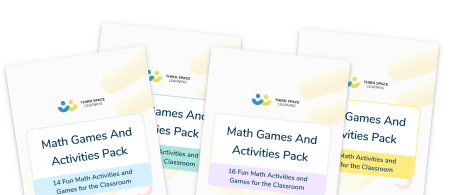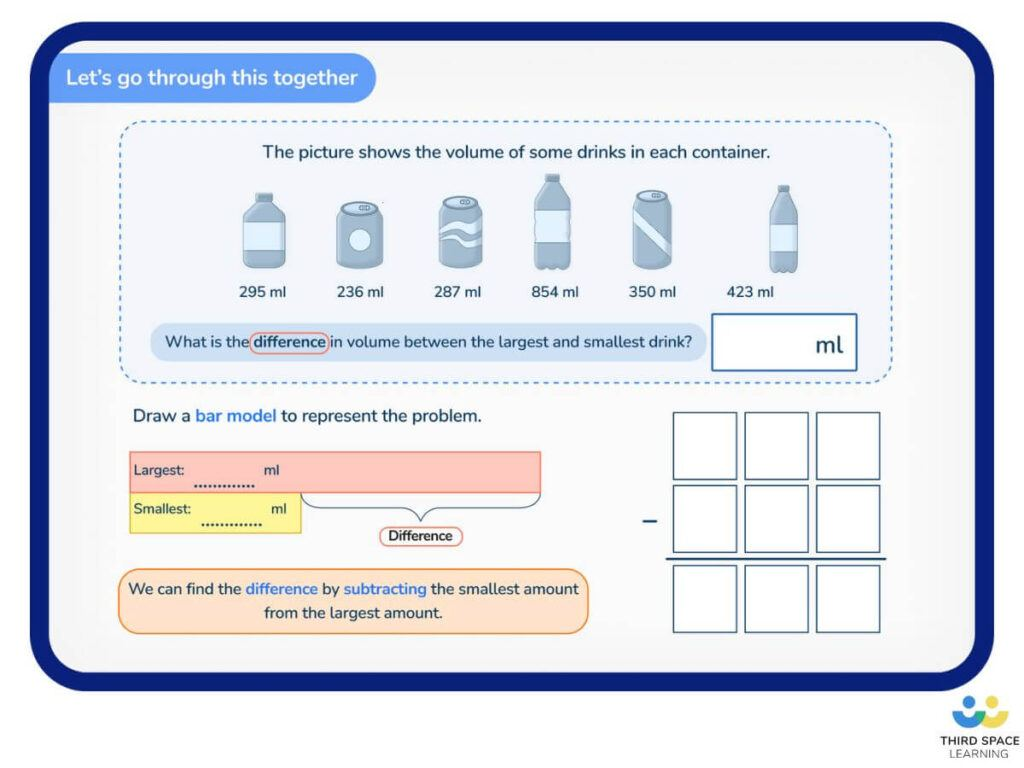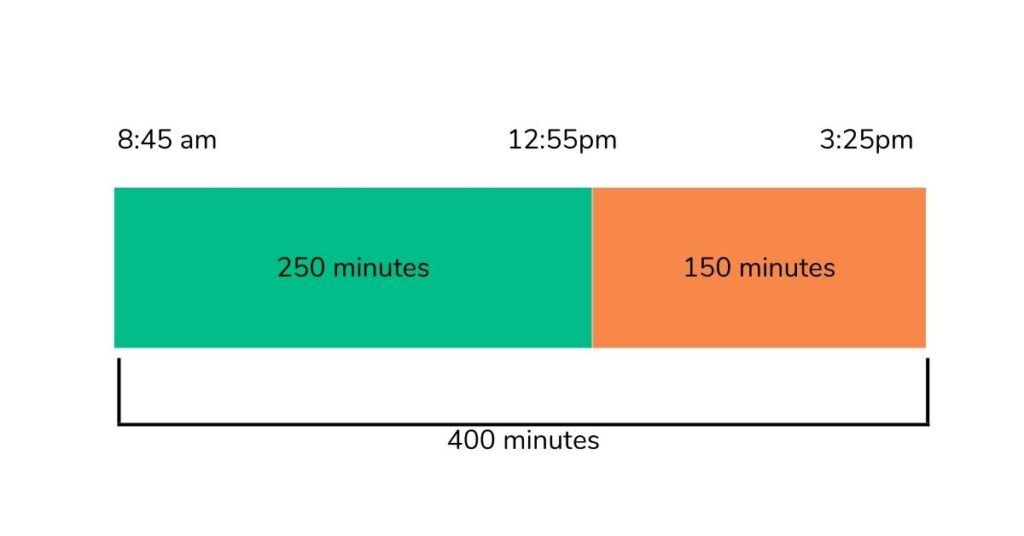Math teaching support you can trustone-on-one tutoring sessionsschools supported

[FREE] Fun Math Games & Activities Packs

Always on the lookout for fun math games and activities in the classroom? Try our ready-to-go printable packs for students to complete independently or with a partner!

# The Ultimate Guide To The Bar Model: How To Teach It And Use It In Elementary School

Teaching the bar model in elementary school is essential if you want students to do well in their reasoning and problem solving skills. Here’s your step-by-step guide on how to teach the bar model using math mastery lessons from Pre-K and Kindergarten right up to 5th grade level questions.

First we will look at the four operations and a progression of bar model representations that can be applied across all grades in elementary school.

Then we will look at more complex bar model examples, including how to apply bar models to other concepts such as fraction and equations.Fun Math Games and Activities Packs for Kindergarten to 5th Grade

Individual packs for K-5 containing fun math games and activities to do with your studentsDownload Free Now!

### What is a bar model in math?

What is a bar model? In math a bar model, also known as a strip diagram, is a pictorial representation of a problem or concept where bars or boxes are used to represent the known and unknown quantities. Bar models are most often used to solve number problems with the four operations – addition and subtraction, multiplication and division.

In word problems, bar models help children visualize the problem and decide which operations to use.

The bar model is the pictorial stage in the concrete pictorial abstract (CPA) approach to learning and is central to math mastery. Bar models will not, however, do the calculations for the students; they simply make it easier for learners to work out which equation must be done to solve the problem.

#### What is the bar model in math?

The bar model is used by drawing out a bar to represent the known and unknown quantities in a given problem. Encouraging a child to use the bar model in a problem can help them to understand conceptually what math operation is required from the problem, and how each part combines to make the whole.

An understanding of bar models is essential for teaching word problems, especially those using four operations (addition, subtraction, multiplication, division), fractions and algebra. Here the bar model shows us that if 2 rectangles out of 3 rectangles are green, then two thirds of 12 must be 8.

#### Why use the bar model method in math?

The bar model is used in Singapore and Asian Math textbooks and is an essential part of the mastery math approach used by schools at all stages.

By using the bar method to visualize problems, students are able to tackle any kind of number problem or complex word problem.A word problem from Third Space Learning’s online platform showing how to represent ratios with a bar model.

Because bar models only require pencils and paper, they are highly versatile and can be very useful for tests.

The use of bar models are also useful for learning at an early stage, from showing number bonds to ten or partitioning numbers as part of your place value work.

Once a child is secure in their use of the bar model for the four operations and can conceptualize its versatility, they can start to use it to visualize many other math topics and problems, such as statistics and data handling.

#### The Singapore Bar Model Method

‘The Singapore Math Model’ is another name for the bar model method. Despite this name however the Singapore bar model (like most of the math mastery approach) is based heavily on the work of Bruner, Dienes and Bishop about the best way to help children learn: teaching for mastery.

Bar models act as a ‘bridge’ between the concrete, pictorial and abstract (CPA in math); once children are secure with using pictorial versions of their concrete materials, they can progress to using bars as visual representations.

Bars are a more abstract way of representing amounts, making the transition to using wholly abstract numbers significantly less difficult.

#### The Part Whole Method

Also known as the ‘part part whole’ method or the comparison model, this kind of bar model uses rectangles to represent the known and unknown quantities as parts of a whole. This is an excellent method to help students represent the very common ‘missing number’ problems.

This can be done in two ways:

• As discrete parts to a whole – each unit in the problem has its own individual box, similar to using Numicon cubes.
• As continuous parts to a whole – units are grouped into one box for each amount in the problem e.g. in 26 + 52, 26 would have one long bar, not 26 smaller rectangles joined together.An example of a continuous part-whole bar model from the Third Space Learning resource Let’s Practice with Bar Models.

When using the part-whole method, proportionality is key; all the bars must be roughly proportional to each other e.g. 6 should be about twice the length of 3.

Part-whole models are generally used to visually represent the four operations, fractions, measurement, algebra and ratios, but can be applied to many more topics in the common core (as long as they’re relevant!).

Read more: What Is The Part Whole Model?

### How to draw a bar model

There are a few steps involved in drawing a bar model and using it to solve a problem:

2. Circle the important information
3. Determine the variables: who? what?
4. Make a plan for solving the problem: what operation needs to be used?
5. Draw the unit bars based on the information
6. Re-read the problem to make sure that the bar models match the information given
7. Complete the problem using the determined operation.

### Bar models: Pre-K to third grade

Students in Pre-K and Kindergarten will routinely come across expressions such as 4+3.

Often, these expressions will be presented as word problems: Aliya has 4 oranges. Alfie has 3 oranges. How many oranges are there altogether? To help children fully understand later stages of the bar model, it is crucial they begin with concrete representations.

There are 2 models that can be used to represent addition:

Once they are used to the format and able to represent word problems with models in this way themselves (assigning ‘labels’ verbally), the next stage is to replace the ‘real’ objects with objects that represent what is being discussed (in this case, we replace the ‘real’ oranges with button counters):

The next stage is to move away from the concrete to the pictorial. As with all the stages, when students are ready for the next stage is a judgment call that is best decided upon within your school.

A general rule of thumb would be that towards the end of kindergarten or start of first grade, students should be able to understand and represent simple addition and subtraction problems pictorially and assign written labels in a bar model.

The penultimate stage is to represent each object as part of a bar, in preparation for the final stage:

The final stage moves away from the 1:1 representation. Each quantity is represented approximately as a rectangular bar:

As mentioned before, it is a judgment call for your school to make, but if you want students to use the bar model to support them at the end of semester tests towards the later stage of their early years, they are going to need to have had a fair amount of experience in this final stage.

#### Bar model subtraction

The same concrete to pictorial stages can be applied to subtraction. However, whereas with addition it is really down to the student’s preference as to which of the 2 bar representations to use, with subtraction the teacher can nudge students to one or the other.

The reason? One represents a ‘part-part-whole’ model, the other a ‘find the difference’ model. Each will be more suited to different word problems and different learners. Let’s examine those at the final stage of the bar model:

Part-part-whole

Austin has 18 lego bricks. He used 15 pieces to build a small car. How many pieces does he have left?

Equation: 18 – 15 =

Find the difference

Austin has 18 lego bricks. Lionel has 3 lego bricks. How many more lego bricks does Austin have than Lionel?

Equation: 18 – 3 =

#### Bar model multiplication

Bar model multiplication starts with the same ‘real’ and ‘representative counters’ stages as addition and subtraction. Then it moves to its final stage, drawing rectangular bars to represent each group:

#### Bar model division

Due to the complexity of division, it is recommended to keep grouping and sharing until the final stage of the bar model is understood. Then word problems such as the 2 below can be introduced:

Sharing

Grace has 27 lollipops. She wants to share them amongst 9 friends by putting them in party bags. How many lollipops will go into each party bag?

Grouping

Grace has 27 lollipops for her party friends. She wants each friend to have 3 lollipops. How many friends can she invite to her party?

#### Bar models in assessments

The key question at any grade level is, “what do we know?” By training students to ask this when presented with word problems themselves, they quickly become independent at drawing bar models.

For example, in the problem: Egg boxes can hold 6 eggs. We need to fill 7 boxes. How many eggs will we need?

We know that there will be 7 egg boxes, so we know we can draw 7 rectangular bars. We know that each box holds 6 eggs, so we can write ‘6 eggs’ or ‘6’ in each of those 7 rectangular bars. We know we need to find the amount of eggs we have altogether. We can see we will need to use repeated addition or multiplication to solve the problem.

#### Bar model for the four operations word problems

Let’s ramp up the difficulty a little. In a sample assessment, students are asked:

A bag of 5 lemons costs $1. A bag of 4 oranges costs$1.80. How much more does one orange cost than one lemon?

Students could represent this problem in the below bar model, simply by asking and answering ‘what do we know?’

From here it should be straightforward for the students to ‘see’ or visualize their next step. Namely, dividing $1.80 by 4 and$1 by 5. Some students will not need the bar model to represent the next stage, but if they do, they would solve and then allocate the cost onto the model:

Then those students that needed this stage, should be able to see that to answer the question, they need to solve 45¢ – 20¢. With the answer of 25¢.

#### Bar model for word problems with fractions

Here’s another example from tests involving fractions and how it can be solved using a fraction bar model

On Saturday Lara read two fifths of her book. On Sunday, she read the other 90 pages to finish the book. How many pages are there in Lara’s book? If we create our bar model for what we know:

Students will then see that they can divide 90 by 3:

As fractions are ‘equal parts’ – a concept they should be familiar with from third grade – they know that the other 2 fifths (Saturday’s reading) will be 30 pages each:

Then they can solve 30 x 5 = 150

### Bar models: Middle school and beyond

#### Equations with the bar model

There are lots of other areas where bar models can assist student’s understanding such as ratio, percentages and equations. In this final example, we look at how an equation can be demystified using the comparison model:

2a + 7 = a + 11

Let’s draw what we know in a comparison model, as we know both sides of the equation will equal the same total:

The bars showing 7 and 11 could have been a lot smaller or larger as we don’t know their relative value to ‘a’ at this stage. However, it is crucial that the ‘a’ appearing first in both bars is understood to be equal (even if it is only approximately equal when drawn freehand in the bar). This allows the student to ‘see’ that to work out the second ‘a’ in the top bar, they can solve 11-7.

So if that ‘a’ is 4, then both the other ‘a’s will also be 4. So each side of the equation will total 15. The below model shows all sections completed. This is not necessary for the students to do, the representation is merely useful until they can see the steps necessary to solve whatever they are faced with:

Where next?

Now you’re persuaded (we hope!) that the bar model in math is going to revolutionize problem solving across your school from early years onwards.

If you don’t believe me, use this problem to grab their attention:

With or without bar models? Which is easier? My guess is your staff will be hooked!

More on the bar model and math mastery techniques

Do you have students who need extra support in math?
Give your students more opportunities to consolidate learning and practice skills through personalized math tutoring with their own dedicated online math tutor.

Each student receives differentiated instruction designed to close their individual learning gaps, and scaffolded learning ensures every student learns at the right pace. Lessons are aligned with your state’s standards and assessments, plus you’ll receive regular reports every step of the way.

Personalized one-on-one math tutoring programs are available for:

The content in this article was originally written by primary school deputy head teacher Pete Richardson and has since been revised and adapted for US schools by elementary math teacher Jaclyn Wassell.##### Pete Richardson
Walton Le Dale Primary School
Pete is a passionate convert to a mastery approach, blending the best of Singapore, Shanghai & home grown pedagogy. He blogs for Third Space on Mastery, & more!
x#### Fun Math Games and Activities Packs for Kindergarten to 5th Grade

Individual packs for K-5 containing fun math games and activities to do with your students

#### Fun Math Games and Activities Packs for Kindergarten to 5th GradeIndividual packs for K-5 containing fun math games and activities to do with your students